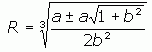SEARCH HOMEMath Central Quandaries & QueriesQuestion from Farzan, a student: Equation 1 : (R + (6.67*5.98*10^13/((R^2)*2)))^2 - (R^2) = (7.27*(10^-5)*R)^2 Equation 2 : (6.67*5.98*10^13/(R^2*2))^2 + 6.67*5.98*10^13/R = (7.27*10^-5*R)^2 As you see if we expand the left part of the first equation and simplify, the equations become same, but these two equations have different answers in my math software.The first one has 3 amounts for R, but the second one has 6 amounts. Why are the answers different ?Farzan,

I get two answers, not three or six. What are the values you get? Are some close? That could be a rounding/approximation limitation in the software.

Refer again to your earlier question. You can use the quadratic formula to solve for x, and thence for R. I get the following expression using the same variables as in the earlier message:Thus, that ± will create two values for R.

Cheers,
Stephen La Rocque.Math Central is supported by the University of Regina and The Pacific Institute for the Mathematical Sciences.